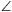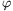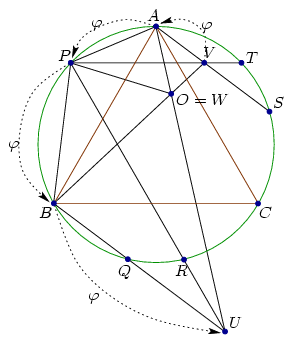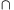Mathematical and Physical Journal
for High Schools
Issued by the MATFUND Foundation
 Already signed up? New to KöMaL?

#Problem A. 438. (November 2007)

A. 438. On the circumcircle of the equilateral triangle ABC, choose an arbitrary point P on arc AB. Mark the points Q and R on arc BC and the points S and T on arc CA for which AP=BQ=CR=CS=AT holds. Let lines BQ and PR meet at U and let lines AS and PT meet at V. Finally, let lines AU and BV meet at W. Show that each two of lines AU, BV and PW enclose an angle of 60o.

(5 pont)

Deadline expired on December 17, 2007.

Solution (sketch). Triangles APV and PBA are similar sinceVAP=SAP=APB=PBQ=PBU=120o andAPV=PBA.

Letbe the rotated homothety which transforms triangle APV into triangle PBA, i.e.(V)=A,(A)=P and(P)=B. The angle between vectors AP and PB is 60o, so the angle of rotation is 60o.The image of line PB is BQ, because(PB) passes through(P)=B and its direction matches the direction of BP (sincePBQ=120o). It can be obtained similarly that(AB)=PR. Then(B)=(PBAB)=(PB)(AB)=BQPR=U.

Therefore transformationmoves V to A, A to P, P to B, and B to U.

Let W be the homothety center. Then AOV=POA=BOP=UOB=60o. So O=W and the lines VW, AW, PW, BW and UW enclose angles of 60o.

### Statistics:

 9 students sent a solution. 5 points: Huszár Kristóf, Korándi Dániel, Kornis Kristóf, Lovász László Miklós, Nagy 235 János, Nagy 314 Dániel, Tomon István, Wolosz János. Unfair, not evaluated: 1 solutions.

Problems in Mathematics of KöMaL, November 2007